calmer than you are

# control flow in brainfuck

Mats Linander
2016-01-14 - New York

Imperative programming languages normally offer developers a multitude of control flow statements. We are accustomed to using loops like for and while; conditional branches like if and switch; subroutine invocations and perhaps even unconditional jumps like goto. Similarly, there is usually a plethora of arithmetic operators that make comparing things a breeze.

True to its name, brainfuck is markedly different. Its only control flow statement also doubles as its only means of performing arithmetic comparison. In this blog post, we’ll have a look at some common high level control flow mechanisms and how the equivalent functionality can be implemented in brainfuck.

The reader is encouraged to read up a bit about brainfuck before proceeding, for instance by checking out the first half or so of this previous post, but no prior experience of the language should really be needed.

# what we have to work with

Brainfuck’s [ and ] instructions are functionally equivalent to a “while non-zero” loop, i.e. a while loop that keeps iterating until some variable reaches 0. If the variable is zero at the beginning of the loop, then the loop will never be entered. A brainfuck program like this:

[]


Is roughly equivalent to:

while (x != 0) {
}


Programming with “while non-zero” as the only conditional statement and as the only means of arithmetic comparison can be a bit of a challenge. However, as the rest of this post hopefully illustrates, mastering a handful of relatively simple brainfuck idioms can go a long way.

# if non-zero (destructively)

Let’s say we wish to write a program like this:

x = read()
if (x != 0) {
write(x)
}


Accomplishing the same in brainfuck, using only “while non-zero”, is not too difficult: replace the if (x != 0) with a while (x != 0) and then make sure x == 0 before the loop is able to iterate a second time. Brainfuck doesn’t have an assignment instruction, so clearing x requires a nested while loop:

x = read()
while (x != 0) {
write(x)
while (x != 0) {
x = x - 1
}
}


At first glance this program may appear clunky and strange, but each of the seven lines can be translated directly to a brainfuck instruction. The result is as elegant as it is terse:

,[.[-]]


Let’s walk through the code. Input is read into a memory cell using the , instruction. A “while non-zero” loop is opened using [ and the . instruction writes the memory cell as output. The inner loop [-] repeatedly decrements the memory cell until it reaches zero, which allows execution to move past the final ].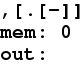The animation above shows the program being executed with the number 4 provided as input. At each step we can see the currently executing instruction highlighted in red and how it affects the memory cell. If the number 0 was read instead, then the outer loop wouldn’t be entered and no output would be written. Execution would flow like this: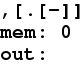To summarize, if (x != 0) { stuff } can be implemented in brainfuck like this: [ stuff [-]]. Albeit in a way that destroys the value held in x.

# if non-zero (non-destructively)

So what if we need to retain the byte read as input? Well, x itself definitely needs to be cleared for the “while non-zero” loop to terminate, but there’s no reason we can’t make a copy of it in the process.

y = 0
while (x != 0) {
write(x)
while (x != 0) {
x = x - 1
y = y + 1
}
}


We introduce a new variable y and initialize it to 0. The clear loop has been modified so that each time x is decremented, y is also incremented. Our clear loop has been turned into a move loop. However, brainfuck doesn’t really have variables, so the pseudo code can’t just be translated line by line as we did in the destructive case.

What brainfuck does have though is a large, contiguous memory area and a pointer pointing into the memory area. Initially, all memory cells are set to 0 and the pointer points at the leftmost cell. Brainfuck is all about moving that pointer around and operating on the cell it points at.

Using the < and > instructions, we can step the pointer left and right respectively. This allows us to make use of a second memory cell in place of the variable y. In this case, we use the cell to the right of where we store x:

,[.[->+<]]


Notice that the only difference from the previous (destructive) “if non-zero” is that we’ve replaced [-] with [->+<]. Instead of clearing x, we move it.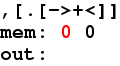More generally, we can implement if (x != 0) { stuff } non-destructively like this: [ stuff [->+<]]. Beware that stuff must be kept from tampering with both the current cell and the one above it, since these are both accessed in the move loop.

# if zero

At this point we’ve familiarized ourselves with two ways of checking if something is non-zero. What about the opposite? How do we check if (x == 0)? Well, here’s one way:

y = 1
if (x != 0) {
y = y - 1
}
if (y != 0) {
stuff
}


The variable y holds a flag that gets cleared if and only if x != 0. In other words, if x == 0 then and only then will the flag remain set. Very simple stuff both in pseudo code and in brainfuck. For the latter, we will of course have to use one of our “if non-zero” constructs in place of the pseudo code’s if statements.

>+<,            # set flag and read x
[>-<[-]]        # if x non zero then clear flag
>[ stuff -]     # if flag still set then do stuff


The following two animations visualize the execution of such a program, where stuff writes the flag as output if x == 0. First with 0 as input: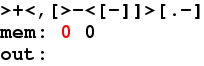And then with 4 as input: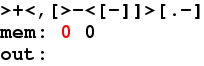Note that while we chose to use the destructive “if non-zero” here, we could just as well have used the non-destructive one.

# if zero (non-destructively, efficiently)

An issue with the approaches we’ve seen so far, destructive and non-destructive alike, is that they can be rather slow. Due to the clear or move loops involved, the run time of the code will be proportional to the value we’re checking. E.g., if that x happens to be 155 then it will take 155 iterations before the loops terminate.

We can do better:

>+<,[>-]>[>]<[ stuff -]


This approach is more complex than what we’ve seen previously. Instead of nice, balanced loops, where the number of < is equal to the number of >, we have loops like [>-] that repositions the memory pointer if entered. These conditionals are required to guarantee that the pointer ends up in the same place, regardless of whether the value inspected was zero or not.

Let’s say the byte read is non-zero. The [>-]> will then clear the flag and reposition the pointer to the cell above it. Since this cell holds 0, the following [>] won’t be executed and the final < repositions the pointer back to the flag cell, which will still be 0. Consequently, if the byte read was non-zero, then stuff will not be executed.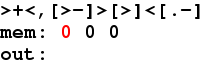Say the byte read was zero. This time [>-] won’t be entered, which leaves the flag cell set to 1. The [>] will be now be entered and therefore reposition the pointer to the empty cell above the flag. The final < moves the pointer back to the flag cell, which will still be 1. Consequently, if the byte read was zero, then stuff will be executed.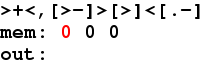# if equal

So why have we spent so much time devising these checks for something being zero and non-zero? What is it all good for? Well, brainfuck doesn’t have any instructions for checking if one thing equals another, but we can use the arithmetic instructions and “if zero” to the same effect. If x == A, where A is some constant, then it must also hold that x - A == 0.

Say we wish to write if (x == 4) { stuff }. Here’s how:

>+<,                  # set a flag and read x
----                  # subtract 4 from x
[>-]>[>]<[ stuff -]   # if x became 0 then do stuff
<++++[-]              # restore and clear x


Notice how we took care to restore x by adding 4, even though we then immediately clear it with a [-]. This is strictly speaking not required in this tiny program, but it does illustrate an important point. Here’s an example of the program executing with the number 2 provided as input: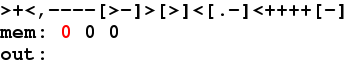In most brainfuck environments, including the one executing in the animation, cells are 8 bit unsigned integers. Subtracting from 0 means the value will wrap around to 255, so subtracting 2 from 0 results in the first cell holding 254. Clearing that value with a [-] loop would take 254 iterations in our case, but in other brainfuck environments it may take many, many more. For instance, if cells were 32 bits, then we’d be looking at more than 4 billion iterations which would severely impact the performance of the program. Portable brainfuck code recognizes this issue and always makes sure to restore variables before iterating over them.

Here’s what the computation looks like when the number 4 is read and the equality condition is met: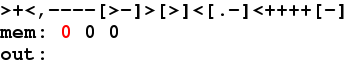# switch

The final mechanism we’ll consider is the switch statement. It is a little bit redundant, since the same functionality can be achieved with a sequence of “if equal”, but it is still good to be familiar with it.

x = read()
switch (x) {
case 6 {
foo
}
case 5 {
bar
}
case 2 {
baz
}
}


The brainfuck version has three levels of nested loops, each of which preceded by subtractions matching one of the three cases. These loops are entered unless the corresponding case is matched. If no case matches then the innermost loop will clear the value, and more significantly also a flag. The last three lines each check the flag, execute their code. The last three lines can trust that if the flag is still set then their case has been matched.

+>,
--[---[-[<->+++++[-]]
<[- foo ]>]
<[- bar ]>]
<[- baz ]


The following two animations visualize this procedure for values 2 and

1. Here we’ve replaced foo, bar and baz with ..., .. and . respectively.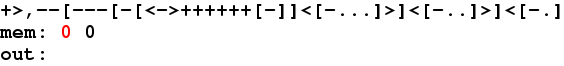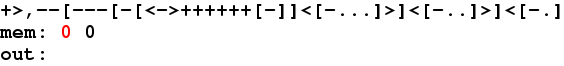Again, if none of the cases matches then the flag will be cleared by the inner loop, so no output will be produced. Here’s the program executing with 8 as input.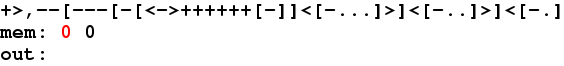Making this construct non-destructive is not too difficult, but it is left as an exercise for the reader.

# Summary

Developing in brainfuck can be a daunting experience. Especially so due to the limited options for control flow. As we’ve seen in this post, there are mechanisms and idioms that cover most of what one would expect from a high level language.

With that said, brainfuck is still a very special language and one shouldn’t expect to be able to apply this material blindly. Writing a non-trivial brainfuck program is a challenging task and requires genuine understanding. We hope this post has made things a little bit clearer.

That was all.